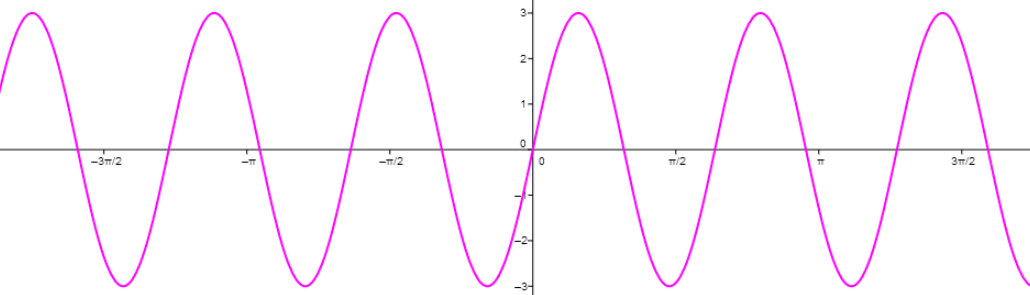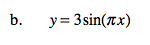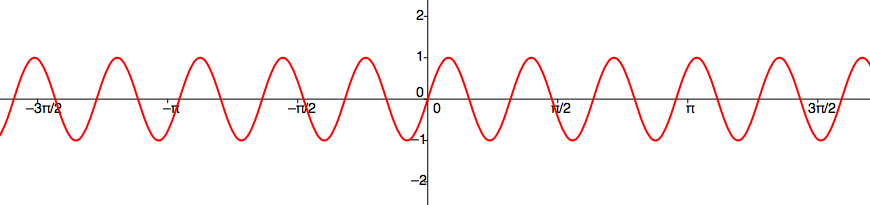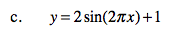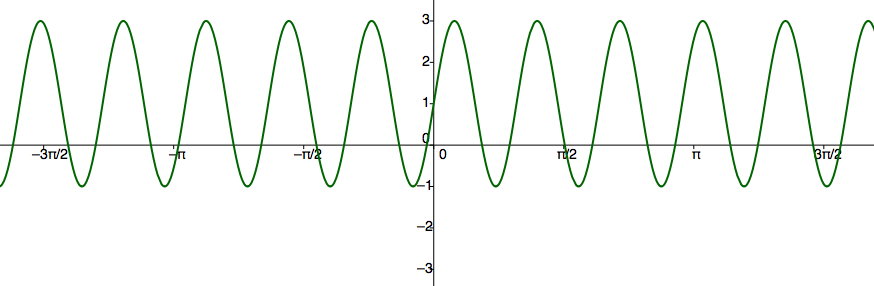Home > CCA2 > Chapter 8 > Lesson 8.1.1 > Problem8-14

8-14.
1. Sketch the graph of each equation below. Homework Help ✎

1. y = sin(2πx)

2. y = 3sin(πx)

3. y = 2sin(2πx) + 1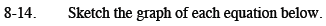For each of the following, determine the amplitude, period, and vertical shift.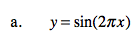This is the graph of y = sin x with a changed period.